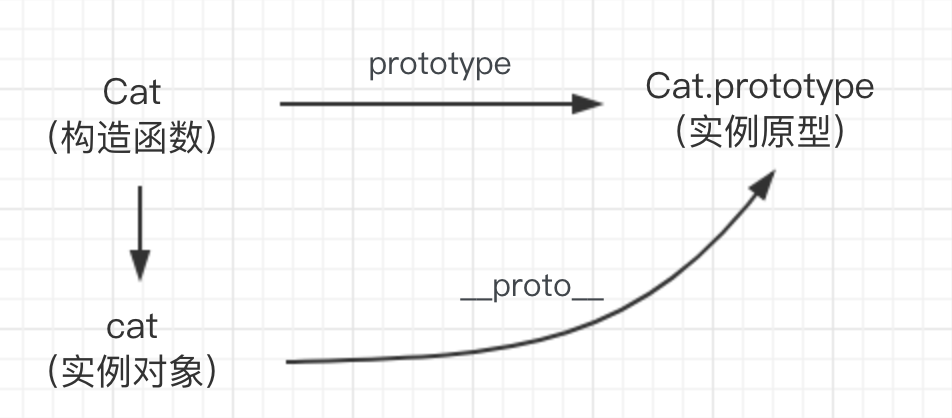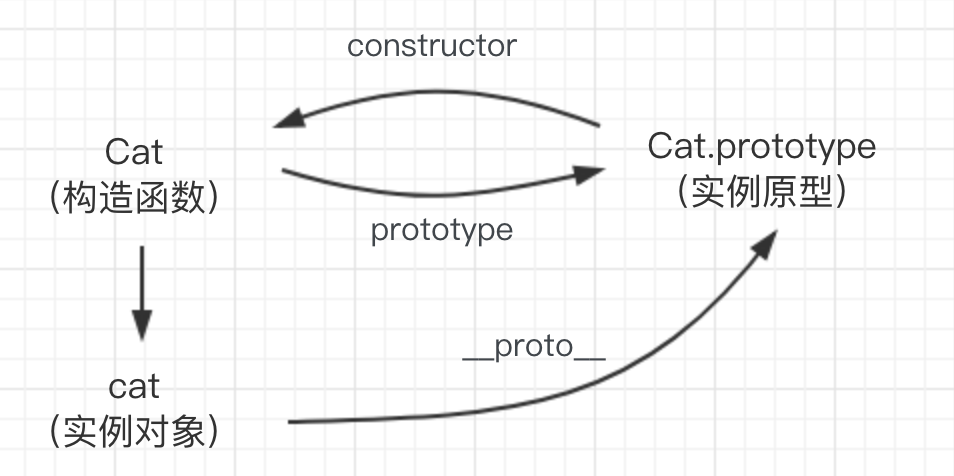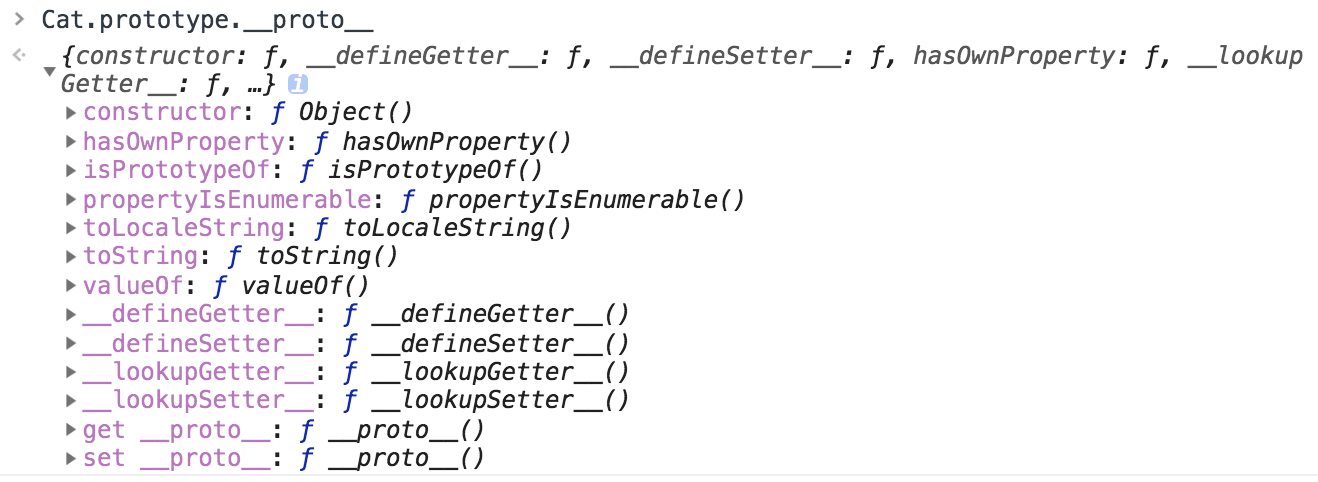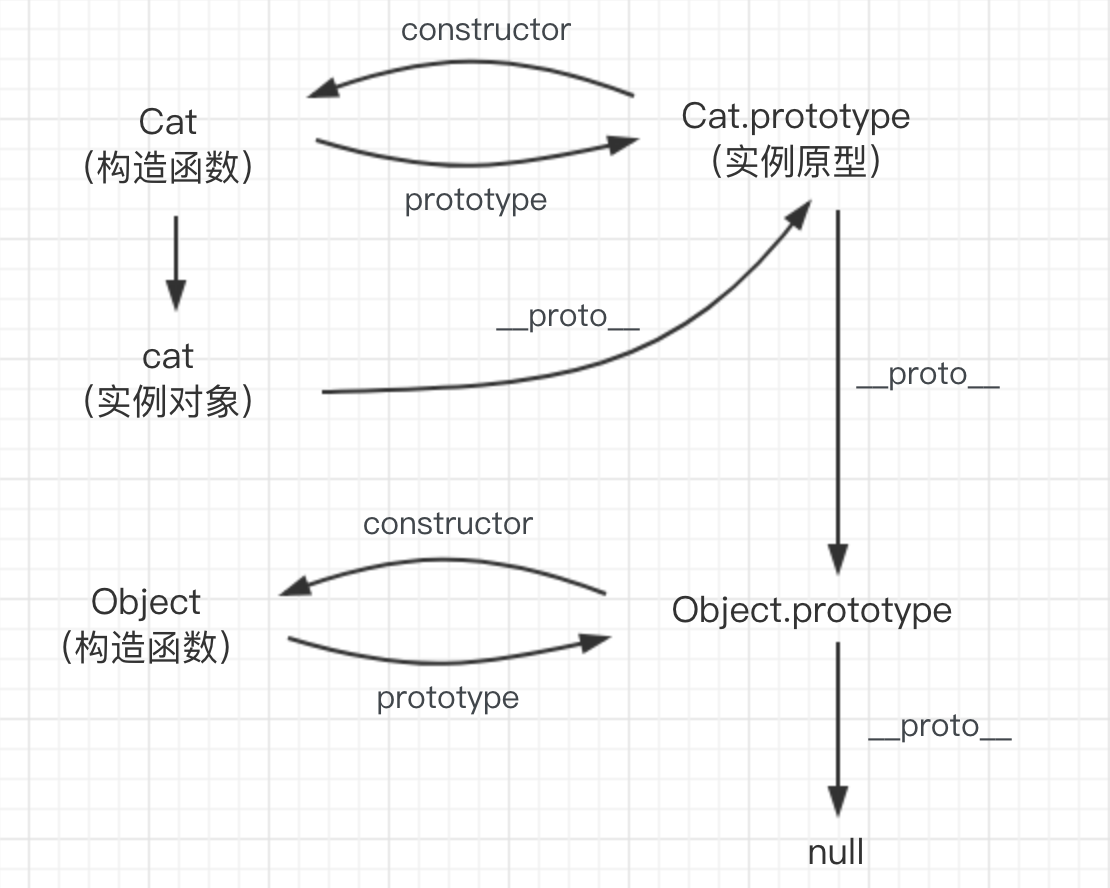JavaScript 也是一门面向对象的语言，很神奇的是，在 ES6 之前，JavaScript 中没有 class 语法。接触过大学课程的都知道，c++ 也是面向对象的，典型的面向对象语言都是通过类来创建实例对象。
``````// c++ 通过类创建对象
class Cat {
public:
string color
}

Cat *cat = new Cat()
``````

### 构造函数

``````function Cat() {
this.color = 'orange'
}

var cat = new Cat()
console.log(cat.color)     // orange
``````

### prototype

``````function Cat() {
this.color = 'orange'
}

console.log(Cat.prototype)
``````（Cat.prototype 在控制台的输出结果）

### __proto__

``````function Cat() {
this.color = 'orange'
}

var cat = new Cat()
console.log(cat.__proto__)
console.log(Object.getPrototypeOf(cat) === cat.__proto__)  // true
``````（cat.__proto__ 在控制台的输出结果）

``````function Cat() {
this.color = 'orange'
}

var cat = new Cat()

console.log(cat.__proto__ === Cat.prototype)   // true
``````（构造函数的 prototype 和实例对象的 __proto__ 关系图）

### constructor

``````function Cat() {
this.color = 'orange'
}

console.log(Cat.prototype.constructor === Cat)    // true
``````（实例原型的 constructor 属性指向相关构造函数）

### 原型链

``````function Cat() {
this.color = 'orange'
}

Cat.prototype.age = 4

var cat = new Cat()

console.log(cat.color)    // orange
console.log(cat.age)      // 4
``````

``````function Cat() {
this.color = 'orange'
}

var cat = new Cat()

console.log(Cat.prototype.__ptoto__)
``````（构造函数 Cat 实例原型的原型）

``````function Cat() {
this.color = 'orange'
}

console.log(Cat.prototype.__ptoto__ === Object.prototype)    // true
``````

``````console.log(Object.prototype.__proto__)   // null
``````（原型链）

### 关于继承

JavaScript 的继承是基于原型链的，在原型链的任何位置设置属性，都能被对象访问到，原型的作用也是在此，它可以包含所有实例共享的属性和方法，就像该属性本来就在实例对象上一样，与其说是继承，不如说原型链建立了一个链条，可以顺藤摸瓜，实例对象可以访问这根链条上的属性和方法。
``````function Cat() {
this.color = 'orange'
this.age = 4
}

Cat.prototype.getColor = function() {
console.log(this.color)
}

Object.prototype.getAge = function() {
console.log(this.age)
}

var cat = new Cat()

cat.getColor()       // orange
cat.getAge()
``````

``````var a = ['hello', 'world']
function f() {}

console.log(a.__proto__ === Array.prototype)      // true
console.log(f.__proto__ === Function.prototype)   // true
``````

### 小结

• 与原型相关的 prototype 和 __proto__ 分别是什么；
• construct 指向了哪里；
• 原型链和继承。